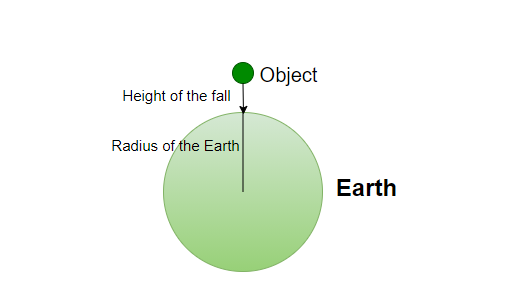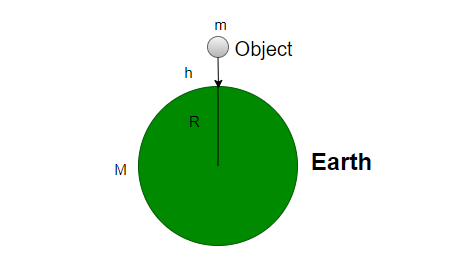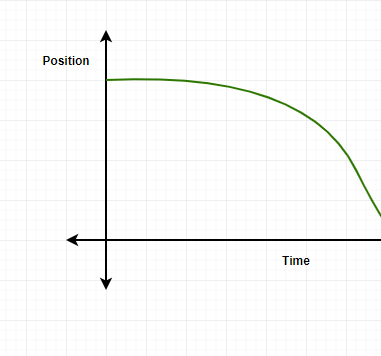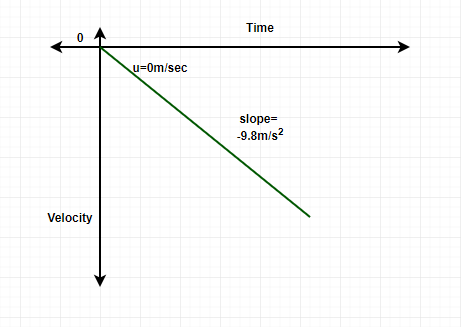Open in App
Not now

# Free Fall

• Last Updated : 12 May, 2021

There are always two cases of things falling to the ground. One is when something is thrown to the ground, for example, throwing a ball. While the other case is when something is dropped to the ground, For example, dropping the ball or accidentally dropping the phone from hands (It will break for sure). These two cases are evidence of the effect of gravity discovered by Sir Isaac Newton.

### What is Free Fall?

Free Fall is defined as the movement of an object under only the influence of Gravity (Gravitational force offered by the planet Earth). Imagine an object being dropped to the ground (Earth’s surface), and no other factor, but the acceleration provided by gravity is acting upon the object, such a case is known as free-falling.### Characteristics of the object under Free Fall

• When the object is under Free Fall, The Initial velocity (u) is always zero, since there is no force acting on the object just when it is dropped. Therefore, u = 0.
• Under Free Fall, No other force than the acceleration due to gravity acts upon the object, which means, even air friction or air resistance is also assumed to be zero.
• The objects start to experience a constant acceleration which is equal to the acceleration due to gravity (g= 9.8 m/sec2).

### Acceleration due to gravity

It is the acceleration offered by the centre of the Earth. The value of acceleration due to gravity is a Universal constant and the value is equal to 9.8 m/sec2. When a body only experiences acceleration due to gravity, that means, it is constantly increasing its velocity with 9.8 m/sec every second starting with a zero velocity.

### Force during Free Fall

The force during Free fall is nothing but the force of Gravitation acting between the object and Earth. While Solving for the Force acting between the object and earth, the height at which the object is dropped is neglected since it has no value as compared to the radius of the earth (R = 6378 km).Force of Gravitation ⇢Since, h<<R

Hence, Height at which the object is dropped can be ignored.Force is defined as, F=ma

Here, a=g (acceleration due to gravity).Where,

G = Gravitational Constant [6.67×10-11 m3kg-1s-2

m = mass of the object

R = Radius of the Earth (6378 km)

### Gravitational Representation of Free Fall

There are majorly two graphs that can be observed of an object under Free Fall. One is the Position-Time graph and the other one is the Velocity-Time graph. The position-time graph will show a curve going down since that object is experiencing acceleration, the starting of the graph will not be curvy since the initial velocity is zero. The velocity-time graph is observed to be having a negative slope since the acceleration is in a downwards (negative) direction.### Newtonian Mechanics (Equation of motion under Free Fall condition)

When a body is in motion whether it is moving in 1-dimension, 2-dimension, projectile, free fall, etc. If it is moving with constant acceleration, The equation of motion by Sir Isaac Newton is applied to the object. There are three formulae or three equation of motion and free fall equation are derived from those 3 basic equations. These are,

First Equation of motion ⇢ v = u+ at

Second equation of motion ⇢ S = ut + 1/2(at2)

Third Equation of motion ⇢ v = u2 + 2as

Condition under Free Fall,

• Initial velocity (u) = 0
• Acceleration = acceleration due to gravity (g)

Hence, the equation obtained are,

1. v = gt
2. h = 1/2(gt2)
3. v = 2gh

### Sample Problems

Question 1: Two objects are dropped together, the other object is 10 times heavier than the first one, while first object falls in 10 seconds, the other takes 12 seconds to fall. Which of the two will obtain more velocity under free fall?

Solution:

Formula for velocity under free fall,

v = gt

Hence, the velocity is independent of the mass or weight.

V1 = 9.8 × 10 = 98 m/sec

V2 = 9.8 × 12 = 117.6 m/sec

Therefore, The second object will have more velocity.

Question 2: What are the three conditions required for the Freefall of an object?

1. There is no other disturbance other than the force of gravitation due to earth.
2. The initial velocity must be zero
3. The object shall fall under a constant acceleration due to gravity.

Question 3: What is the height at which a ball is dropped if it takes 9 seconds to reach the ground. Does the mass of the ball is an important factor in order to obtain the height?

Solution:

The formula for height of the ball,

h = 1/2(gt2)

Since, the formula does not include the mass, hence, the value of mass of the ball is not important in order to find the value of height of the ball.

h = 1/2(9.8× 81)

h = 396.9 meters

Question 4: What was the height of an object when the velocity obtained by the object is 10m/sec?

Solution:

The formula of equation of motion used here,

v= 2gh

10= 2×9.8 × h

h= 0.51 meters

Question 5: What are real-life examples of Free fall?